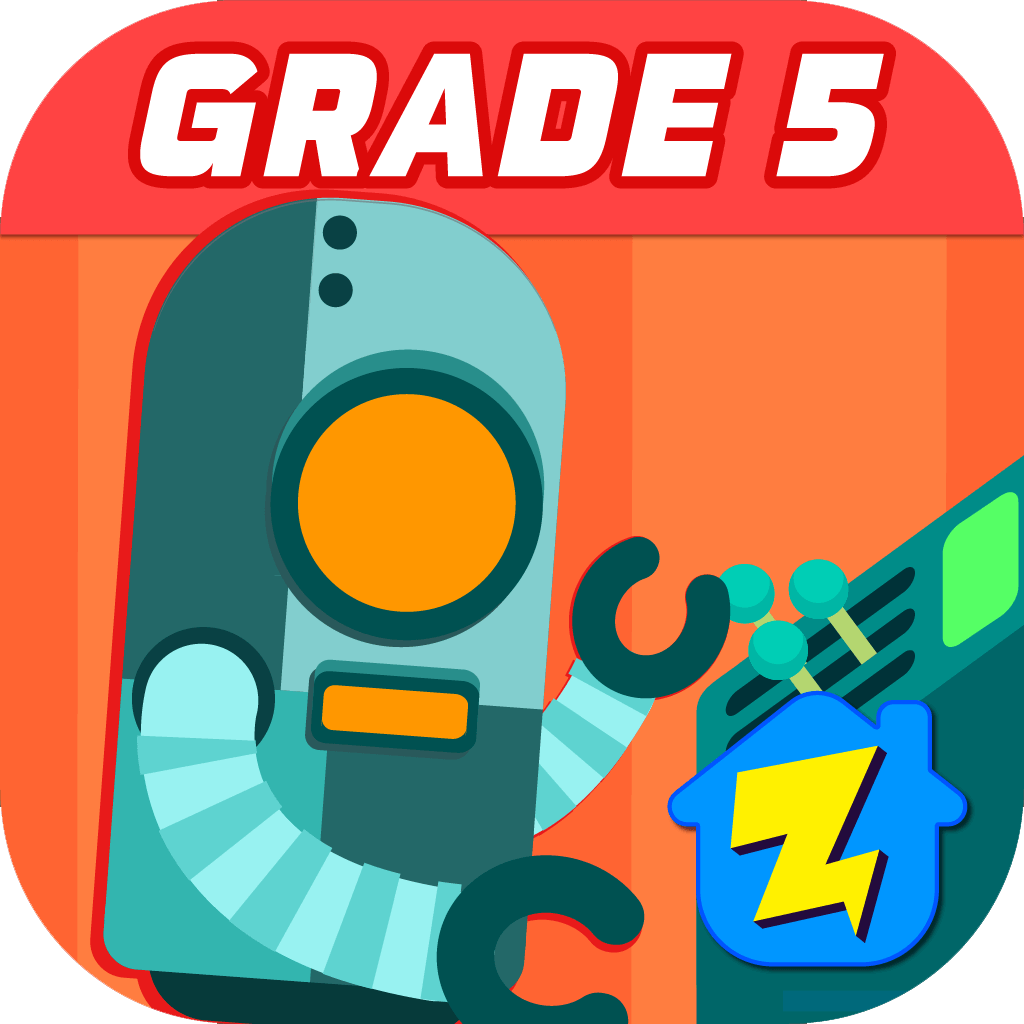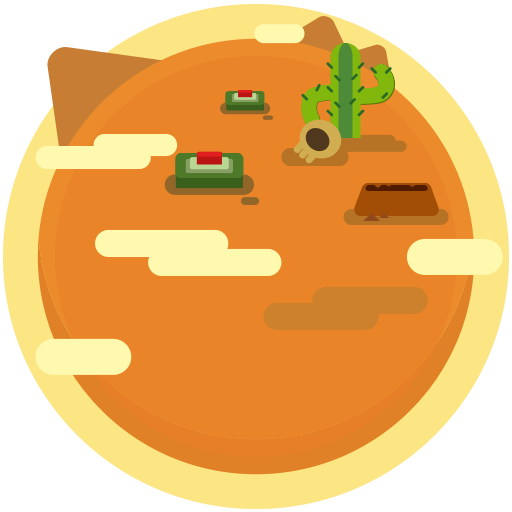## Welcome to Zapzapmath Grade 5 Home App### Players can use this app to learn about math expressions, multiply and divide fractions, convert measurements, understand volume. Graph coordinates, and classify 2D figures.

Our 5th grade math games are tailored according to the needs of students in 5th grade. The games enable the students to have a proper understanding of rounding decimals, multiplying fractions by whole numbers, as well as dividing fractions by whole numbers.

The games are prepared for 5th grade students to improve their knowledge and skills. These skills include rounding of decimals, knowing how to divide fractions by whole numbers, and multiplying fractions by whole numbers.

Topics are offered to help students have a comprehensive knowledge of whole numbers and rounding of decimals, as well as simple strategies for multiplying and dividing fractions by whole numbers.

### Skills that can be learned

##### Multiplying fractions

Knowing how to multiply fractions by fractions

##### Dividing decimals

Knowing how to divide decimals by decimals

##### Rounding decimals

Knowing how to round decimals to any place

### Games Available In This Grade### Rounding Decimals : Franken Wife

Round decimals### Math Fraction Game : Mine Detector

Multiply fractions by fractionsDivide decimals